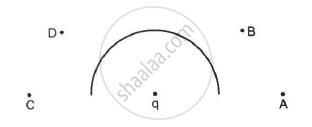Department of Pre-University Education, Karnataka course PUC Karnataka Science Class 12
Share

# In the Following Figure Shows a Charge Q Placed at the Centre of a Hemisphere. a Second Charge Q is Placed at One of the Positions A, B, C and D. - Physics

ConceptElectric Field Introduction of Electric Field

#### Question

In the following figure shows a charge q placed at the centre of a hemisphere. A second charge Q is placed at one of the positions A, B, C and D. In which position(s) of this second charge, the flux of the electric field through the hemisphere remains unchanged?
(a) A
(b) B
(c) C
(d) D#### Solution

(a) A
(c) C
These are the only points in the straight line with the charge q and at the brim of the hemisphere. So, field lines emerging from these charges do not affect the flux through the hemisphere due to charge q. On the other hand, the two remaining charges are beside the surface of the hemisphere and not in line with the charge q and not at the brim. So, they will affect the flux. Hence, the correct answers are (a) and (c).

Is there an error in this question or solution?

#### APPEARS IN

HC Verma Solution for Concepts of Physics - Vol. 2 (2018 to Current)
Chapter 8: Gauss’s Law
MCQ | Q: 6 | Page no. 140
Solution In the Following Figure Shows a Charge Q Placed at the Centre of a Hemisphere. a Second Charge Q is Placed at One of the Positions A, B, C and D. Concept: Electric Field - Introduction of Electric Field.
S